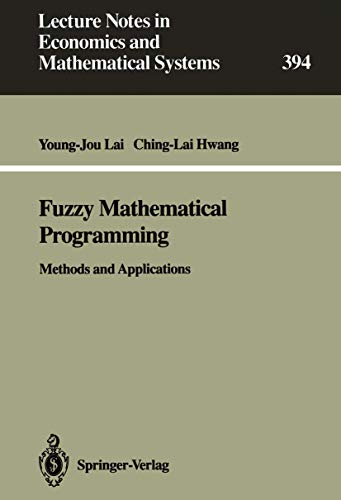Free Shipping in Australia
Proud to be B-Corp

# Fuzzy Mathematical Programming Young-Jou Lai\$205,49
Condition - New
Only 2 left

## Summary

To improve flexibility and robustness of fuzzymathematical programming techniques, we also present ourexpert decision-making support system IFLP which considersand solves all possibilities of a specific domain of (fuzzy)linear programming problems.

## Fuzzy Mathematical Programming Summary

### Fuzzy Mathematical Programming: Methods and Applications by Young-Jou Lai

In the last 25 years, the fuzzy set theory has been applied in many disciplines such as operations research, management science, control theory,artificial intelligence/expert system, etc. In this volume, methods and applications of fuzzy mathematical programming and possibilistic mathematical programming are first systematically and thoroughly reviewed and classified. This state-of-the-art survey provides readers with a capsule look into the existing methods, and their characteristics and applicability to analysis of fuzzy and possibilistic programming problems. To realize practical fuzzy modelling, we present solutions for real-world problems including production/manufacturing, transportation, assignment, game, environmental management, resource allocation, project investment, banking/finance, and agricultural economics. To improve flexibility and robustness of fuzzy mathematical programming techniques, we also present our expert decision-making support system IFLP which considers and solves all possibilities of a specific domain of (fuzzy) linear programming problems. Basic fuzzy set theories, membership functions, fuzzy decisions, operators and fuzzy arithmetic are introduced with simple numerical examples in aneasy-to-read and easy-to-follow manner. An updated bibliographical listing of 60 books, monographs or conference proceedings, and about 300 selected papers, reports or theses is presented in the end of this study.

1 Introduction.- 1.1 Objectives of This Study.- 1.2 Fuzzy Mathematical Programming Problems.- 1.3 Classification of Fuzzy Mathematical Programming.- 1.4 Applications of Fuzzy Mathematical Programming.- 1.5 Literature Survey.- 2 Fuzzy Set Theory.- 2.1 Fuzzy Sets.- 2.2 Fuzzy Set Theory.- 2.2.1 Basic Terminology and Definition.- 2.2.1.1 Definition of Fuzzy Sets.- 2.2.1.2 Support.- 2.2.1.3 ?-level Set.- 2.2.1.4 Normality.- 2.2.1.5 Convexity and Concavity.- 2.2.1.6 Extension Principle.- 2.2.1.7 Compatibility of Extension Principle with ?-cuts.- 2.2.1.8 Relation.- 2.2.1.9 Decomposability.- 2.2.1.10 Decomposition Theorem.- 2.2.1.11 Probability of Fuzzy Events.- 2.2.1.12 Conditional Fuzzy Sets.- 2.2.2 Basic Operations.- 2.2.2.1 Inclusion.- 2.2.2.2 Equality.- 2.2.2.3 Complementation.- 2.2.2.4 Intersection.- 2.2.2.5 Union.- 2.2.2.6 Algebraic Product.- 2.2.2.7 Algebraic Sum.- 2.2.2.8 Difference.- 2.3 Membership Functions.- 2.3.1 A Survey of Functional Forms.- 2.3.2 Examples to Generate Membership Functions.- 2.3.2.1 Distance Approach.- 2.3.2.2 True-Valued Approach.- 2.3.2.3 Payoff Function.- 2.3.2.4 Other Examples.- 2.4 Fuzzy Decision and Operators.- 2.4.1 Fuzzy Decision.- 2.4.2 Max-Min Operator.- 2.4.3 Compensatory Operators.- 2.4.3.1 Numerical Example for Operators.- 2.5 Fuzzy Arithmetic.- 2.5.1 Addition of Fuzzy Numbers.- 2.5.2 Subtraction of Fuzzy Numbers.- 2.5.3 Multiplication of Fuzzy Numbers.- 2.5.4 Division of Fuzzy Numbers.- 2.5.5 Triangular and Trapezoid Fuzzy Numbers.- 2.6 Fuzzy Ranking.- 3 Fuzzy Mathematical Programming.- 3.1 Fuzzy Linear Programming Models.- 3.1.1 Linear Programming Problem with Fuzzy Resources.- 3.1.1.1 Verdegay's Approach.- 3.1.1.1a Example 1: The Knox Production-Mix Selection Problem.- 3.1.1.1b Example 2: A Transportation Problem.- 3.1.1.2 Werners's Approach.- 3.1.1.2a Example 1: The Knox Production-Mix Selection Problem.- 3.1.1.2b Example 2: An Air Pollution Regulation Problem.- 3.1.2 Linear Programming Problem with Fuzzy Resources and Objective.- 3.1.2.1 Zimmermann's Approach.- 3.1.2.1a Example 1: The Knox Production-Mix Selection Problem.- 3.1.2.1b Example 2: A Regional Resource Allocation Problem.- 3.1.2.1c Example 3: A Fuzzy Resource Allocation Problem.- 3.1.2.2 Chanas's Approach.- 3.1.2.2a Example 1: An Optimal System Design Problem.- 3.1.2.2b Example 2: An Aggregate Production Planning Problem.- 3.1.3 Linear Programming Problem with Fuzzy Parameters in the Objective Function.- 3.1.4 Linear Programming with All Fuzzy Coefficients.- 3.1.4.1 Example: A Production Scheduling Problem.- 3.2 Interactive Fuzzy Linear Programming.- 3.2.1 Introduction.- 3.2.2 Discussion of Zimmermann's, Werners's Chanas's and Verdegay's Approaches.- 3.2.3 Interactive Fuzzy Linear Programming - I.- 3.2.3.1 Problem Setting.- 3.2.3.2 The Algorithm of IFLP-I.- 3.2.3.3 Example: The Knox Production-Mix Selection Problem.- 3.2.4 Interactive Fuzzy Linear Programming - II.- 3.2.4.1 The Algorithm of IFLP-II.- 3.3 Some Extensions of Fuzzy Linear Programming Problems.- 3.3.1 Membership Functions.- 3.3.1.1 Example: A Truck Fleet Problem.- 3.3.2 Operators.- 3.3.3 Sensitivity Analysis and Dual Theory.- 3.3.4 Fuzzy Non-Linear Programming.- 3.3.4.1 Example: A Fuzzy Machining Economics Problem.- 3.3.5 Fuzzy Integer Programming.- 3.3.5.1 Fuzzy 0-1 Linear Programming.- 3.3.5.1a Example: A Fuzzy Location Problem.- 4 Possibilistic Programming.- 4.1 Possibilistic Linear Programming Models.- 4.1.1 Linear Programming with Imprecise Resources and Technological Coefficients.- 4.1.1.1 Ramik and Rimanek's Approach.- 4.1.1.1a Example: A Profit Apportionment Problem.- 4.1.1.2 Tanaka, Ichihashi and Asai's Approach.- 4.1.1.3 Dubois's Approach.- 4.1.2 Linear Programming with Imprecise Objective Coefficients.- 4.1.2.1 Lai and Hwang's Approach.- 4.1.2.1a Example: A Winston-Salem Development Management Problem.- 4.1.2.2 Rommelfanger, Hanuscheck and Wolf's Approach.- 4.1.2.3 Delgado, Verdegay and Vila's Approach.- 4.1.3 Linear Programming with Imprecise Objective and Technological Coefficients.- 4.1.4 Linear Programming with Imprecise Coefficients.- 4.1.4.1 Lai and Hwang's Approach.- 4.1.4.2 Buckley's Approach.- 4.1.4.2a Example: A Feed Mix (Diet) Problem.- 4.1.4.3 Negi's Approach.- 4.1.4.4 Fuller's Approach.- 4.1.5 Other Problems.- 4.2 Some Extensions of Possibilistic Linear Programming.- 4.2.1 Linear Programming with Imprecise Coefficients and Fuzzy Inequalities.- 4.2.1a Example: A Fuzzy Matrix Game Problem.- 4.2.2 Linear Programming with Imprecise Objective Coefficients and Fuzzy Resources.- 4.2.2a Example: A Bank Hedging Decision Problem.- 4.2.3 Stochastic Possibilistic Linear Programming.- 4.2.3a Example: A Bank Hedging Decision Problem.- 5 Concluding Remarks.- 5.1 Probability Theory versus Fuzzy Set Theory.- 5.2 Stochastic versus Possibilistic Programming.- 5.3 Future Research.- 5.4 Introduction of the Following Volume.- 5.5 Fuzzy Multiple Attribute Decision Making.- Books, Monographs and Conference Proceedings.- Journal Articles, Technical Reports and Theses.

NLS9783540560982
9783540560982
354056098X
Fuzzy Mathematical Programming: Methods and Applications by Young-Jou Lai
New
Paperback
Springer-Verlag Berlin and Heidelberg GmbH & Co. KG
1992-12-14
306
N/A
Book picture is for illustrative purposes only, actual binding, cover or edition may vary.
This is a new book - be the first to read this copy. With untouched pages and a perfect binding, your brand new copy is ready to be opened for the first time